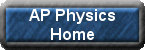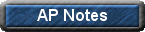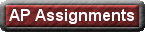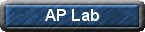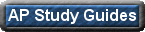AP Physics I Assignments

Unit - Kinematics: Motion in one Dimension

Worksheet 2A - Average Velocity
Worksheet 2B - Acceleration
Worksheet 2C - Displacement with Uniform Acceleration
Worksheet 2D - Velocity and Displacement with Uniform Acceleration
Worksheet 2E - Final Velocity after any Displacement
Worksheet 2F - Falling Objects
Worksheet 3A - Finding Resultant Magnitude and Direction
Worksheet 3B - Resolving Vectors
Worksheet 3C - Adding vectors algebraically

Unit: Newtonian Mechanics and Applying Newton's Laws

Net External Force - 4A
Newton's Second Law - 4B
Coefficients of Friction - 4C
Projectiles launched horizontally - 3D
Projectile Motion with or without an angle - 3F

Unit: Work and Energy

Work - 5A
Kinetic Energy - 5B
Work - Kinetic Energy Theorem - 5C
Potential Energy - 5D
Conservation of Mechanical Energy - 5E
Power - 5F

Unit: Impulse and Linear Momentum

Worksheet 6A - Momentum
Worksheet 6B - Force and Momentum
Worksheet 6C - Stopping Distance
Worksheet  6D - Conservation of Momentum
Worksheet 6E - Perfectly Inelastic Collisions
Worksheet 6F - Kinetic Energy in Perfectly Inelastic Collisions
Worksheet 6G - Elastic Collisions

Unit: Circular Motion and The Law of Universal Gravitation

WS7I: Gravitation
WS7J: Kepler's Law

Unit: Rotational Motion

WS 7A: Angular Displacement
WS 7B: Angular Speed
WS 7C: Angular Acceleration
WS7D: Angular Kinematics
WS7E: Tangential Speed
WS7F: Tangential Acceleration
WS7G: Centripetal Acceleration
WS7H: Centripetal Force

Unit: Extended Bodies at Rest

WS8A: Rotational Equilibrium and Dynamics
WS8B: Rotational Equilibrium
WS8C: Newton's Second Law for Rotation
WS8D: Conservation of Angular Momentum
WS8E: Conservation of Mechanical Energy

Chapter 19: Vibrational Motion

WSA: Hooke's Law
WSB: Simple Harmonic Motion of a Simple Pendulum
WSC: Simple Harmonic Motion of a Spring and Mass System

Chapter 20: Mechanical Waves

WSD: Wave Speed
WS: Intensity of Sound
WSE: Harmonics

Electric Charge, Force and Energy

WS17A: Electric Force - Coulomb's Law - Two Charges
WS17B: Electric Force - Coulomb's Law - More than two charges
WS17C: Equilibrium
WS17D: Electric Field
WS18A: Electric Potential Energy
WS18B: Potential Difference
WS18C: Capacitance

DC Circuits- See Dr. Malik for Assignments

WS19A: Current
WS19B: Resistance
WS19C: Electric Power
WS19D: Cost of Electrical Energy
WS20A: Resistors in Series
WS20B: Resistors in Parallel
WS20C: Resistors in Series and Parallel
WS20D: Current and Potential Difference across a Resistor

Reflection and Refraction of LightDr. Richard MalikPhysics TeacherMarlboro High School, N.J.rmalik@frhsd.com732-617-8393, ext. 6149